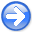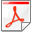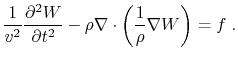Wavefield Seismic Imaging tutorial: exploding reflector'' modeling/migrationNext: Example Up: Sava: WSI tutorial Previous: Sava: WSI tutorial

Introduction

The exploding reflector model, illustrated in Figure 1, allows us to perform zero offset modeling and migration for models of arbitrary complexity (Clærbout, 1985). Under this model, the image is described as a collection of points which explode'', i.e. become sources, at the same time arbitrarily set to be the time origin. Data are obtained at the receivers by forward simulation of acoustic waves from the exploding reflectors. Likewise, images are obtained by backward simulation of acoustic waves from the observed data.

exprefwidth=The exploding reflector model (after Clærbout (1985)).

Acoustic modeling and migration can be implemented using numeric solutions to an acoustic wave-equation, for example a variable-density wave-equation:(1)

In Equation 1,represents the acoustic wavefield,andrepresent the velocity and density of the medium, respectively, andrepresents a source function.
• In modeling, we use the distributed sourceto generate the wavefieldat all positions and all times by wave propagation forward in time. The data represent a subset of the wavefield observed at receivers distributed in the medium:(2)

• In migration, we use the observed datato generate the wavefieldat all positions and all times by wave propagation backward in time. The image represents a subset of the wavefield at time zero:(3)

In both cases, we solve Equation 1 with different initial conditions, but with the same model,andand with the same boundary conditions.Wavefield Seismic Imaging tutorial: exploding reflector'' modeling/migrationNext: Example Up: Sava: WSI tutorial Previous: Sava: WSI tutorial

2014-04-15